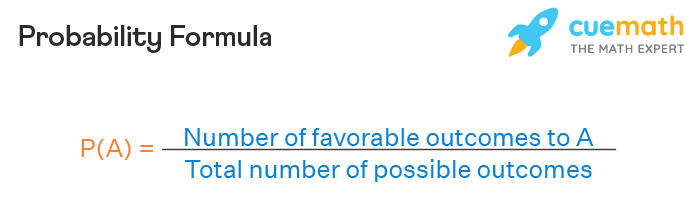# amalhanaja# Random Numbers and Probability

## Introduction

### What are the chances?

Chance (also known as probability) is simply how likely something is to happen.

### Measuring chance### Sampling from a data frame

``````# Sample use np.random.rand
sales_counts.sample() # 1st Attempt. Out: Brian 128
sales_counts.sample() # 2nd Attempt. Out: Claire 75
``````

To ensure the same result when invoking sample function, we should set the random seed then it will generate the same random value each time

``````np.random.rand(10)
sales_count.sample() # 1st Attempt. Out: Brian 128

np.random.rand(10)
sales_counts.sample() # 2nd Attempt. Out: Brian 128

np.random.rand(10)
sales_counts.sample() # 3rd Attempt. Out: Brian 128
``````
``````# Sampling with replacement
sales_counts.sample(5, replace = True)
``````

### Independent Event vs Dependent Event

 Independent Event Dependent Event The probability the next event is not affected by the previous one The probability the next event is affected by the previous one With Replacement Without Replacement

### Calculating probabilities

``````# Count the deals for each product
counts = amir_deals['product'].value_counts()

# Calculate probability of picking a deal with each product
probs = counts / len(amir_deals['product'])
print(probs)
``````

### Sampling deals

``````# Set random seed
np.random.seed(24)

# Sample 5 deals without replacement
sample_without_replacement = amir_deals.sample(5, replace=False)
print(sample_without_replacement)

# Sample 5 deals with replacement
sample_with_replacement = amir_deals.sample(5, replace = True)
print(sample_with_replacement)
``````

## Discrete Distributions

A discrete distribution is a distribution of data in statistics that has discrete values. Discrete values are countable, finite, non-negative integers, such as 1, 10, 15, etc.

### Probability Distributions

Probability distributions describe the probability of each possible outcome in a scenario.

Expected value: mean of probability distributions

We can visualize probability distributions using a bar plot, where each bar represents an outcome, and each bar's height represents the probability of its outcome.

### Law of large number

As the size of your sample increase, the sample mean will approach the expected value.

 Sample Size Mean 10 3.0 100 3.40 1000 3.48

### Creating a probability distribution

``````# 1.
# Create a histogram of restaurant_groups and show plot
restaurant_groups['group_size'].hist(bins=[2,3,4,5,6])
plt.show()

# 2
# Create probability distribution
size_dist = restaurant_groups['group_size'] / len(restaurant_groups)

# Reset index and rename columns
size_dist = size_dist.reset_index()
size_dist.columns = ['group_size', 'prob']

print(size_dist)

# 3
# Expected value
expected_value = np.sum(size_dist['group_size'] * size_dist['prob'])
print(expected_value)

#4
# Subset groups of size 4 or more
groups_4_or_more = size_dist[size_dist['group_size'] >= 4]

# Sum the probabilities of groups_4_or_more
prob_4_or_more = np.sum(groups_4_or_more['prob'])
print(prob_4_or_more)
``````

## Continuous Distribution

### Uniform distribution in Python

``````from scipy.stats import uniform
uniform.cdf(7, 0, 12) # P(wait_time <= 7)
1 - uniform.cdf(7, 0, 12) # P(wait_time >= 7)
uniform.cdf(7, 0, 12) - uniform.cdf(4, 0, 12) # P(4 <= wait_time <= 7)
``````

### Generating random numbers according to uniform distribution

``````from scipy.stats import uniform
uniform.rvs(0, 5, size=10)
``````

### Data backups

``````#1
# Min and max wait times for back-up that happens every 30 min
min_time = 0
max_time = 30

#2
# Calculate probability of waiting less than 5 mins
prob_less_than_5 = uniform.cdf(5, min_time, max_time)
print(prob_less_than_5)

#3
# Calculate probability of waiting more than 5 mins
prob_greater_than_5 = 1 - uniform.cdf(5, min_time, max_time)
print(prob_greater_than_5)

#4
# Calculate probability of waiting 10-20 mins
prob_between_10_and_20 = uniform.cdf(20, min_time, max_time) - uniform.cdf(10, min_time, max_time)
print(prob_between_10_and_20)
``````

### Simulating wait times

``````#1
# Set random seed to 334
np.random.seed(334)

#2
# Import uniform
from scipy.stats import uniform

#3
# Generate 1000 wait times between 0 and 30 mins
wait_times = uniform.rvs(0, 30, size=1000)

print(wait_times)

#4
# Create a histogram of simulated times and show plot
plt.hist(wait_times)
plt.show()
``````

## Binominal Distribution

Describe the probability of the number of successes in a sequence of independent event trials.

Binary Outcome is an outcome of binary value which is 0 and 1.

Expected value = n * p

``````from scipy.stats import binom
binom.rvs(1, 0.5, size=1)
``````
``````# binom.pmf(num heads, num trials, prob of heads)
binom.cdf(7, 10, 0.5) # P(heads <= 7)
1 - binom.cdf(7, 10, 0.5) # P(heads > 7)
``````

### Simulating sales deals

``````#1
# Import binom from scipy.stats
from scipy.stats import binom

# Set random seed to 10
np.random.seed(10)

#2
# Simulate a single deal
print(binom.rvs(1, 0.3, size=1))

#3
# Simulate 1 week of 3 deals
print(binom.rvs(3, 0.3, size=1))

#4
# Simulate 52 weeks of 3 deals
deals = binom.rvs(3, 0.3, size=52)

# Print mean deals won per week
print(np.mean(deals))
``````

### Calculating binominal probabilites

``````#1
# Probability of closing 3 out of 3 deals
prob_3 = binom.pmf(3, 3, 0.3)

print(prob_3)

#2
# Probability of closing <= 1 deal out of 3 deals
prob_less_than_or_equal_1 = binom.cdf(1, 3, 0.3)

print(prob_less_than_or_equal_1)

#3
# Probability of closing > 1 deal out of 3 deals
prob_greater_than_1 = 1 - binom.cdf(1, 3, 0.3)

print(prob_greater_than_1)
``````

# How many sales will be won?

``````# Expected value = n * p

# Expected number won with 30% win rate
won_30pct = 3 * 0.3
print(won_30pct)

# Expected number won with 25% win rate
won_25pct = 3 * 0.25
print(won_25pct)

# Expected number won with 35% win rate
won_35pct = 3 * 0.35
print(won_35pct)
``````